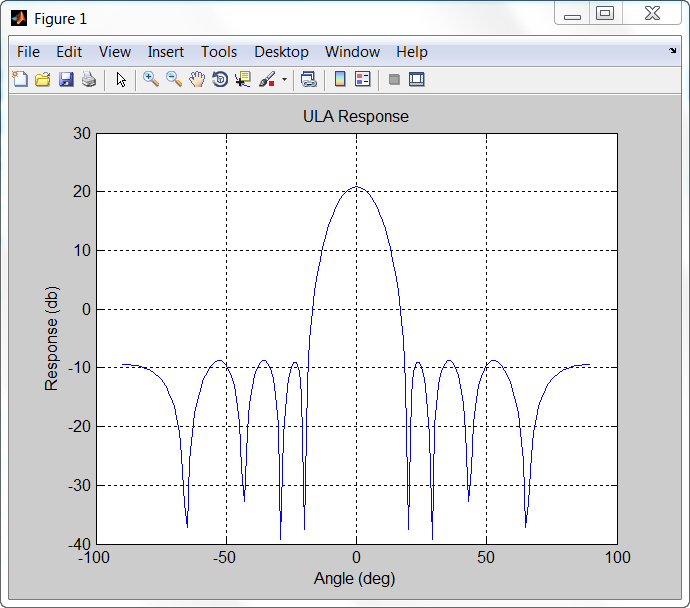Sometimes, it is convenient to put System objects inside a function that is to be called many times. This eliminates the overhead in creating new instances of a System object™ each time the function is called. You can write logic which creates the System object just once and declares it to be `persistent`. For example, suppose you require the response of an 11-element ULA for several different arrival angles and want to plot that response versus angle.
```function plot_ULA_response azangles = [-90:90]; elangles = zeros(size(azangles)); fc = 100e9; c = physconst('LightSpeed'); N = size(azangles,2); lambda = c/fc; d = 0.4*lambda; numelements = 11; resp = zeros(1,N); sIso = phased.IsotropicAntennaElement(... 'FrequencyRange',[1,200]*1e9,... 'BackBaffled',false); sULA = phased.ULA('Element',sIso,... 'NumElements',numelements,... 'ElementSpacing',d,... 'Taper',taylorwin(numelements).'); for n = 1:N x = get_ULA_response(sULA,fc,azangles(n),elangles(n)); resp(n) = abs(x); end plot(azangles,20*log10(resp)); title('ULA Response'); xlabel('Angle (deg)'); ylabel('Response (db)'); grid; end function resp = get_ULA_response(sULA,fc,az,el) persistent sAR; c = physconst('LightSpeed'); if isempty(sAR) sAR = phased.ArrayResponse('SensorArray',sULA,... 'PropagationSpeed',c,... 'WeightsInputPort',false,... 'EnablePolarization',false); end resp = sAR(fc,[az;el]); end```
To create the code, run `codegen` (MATLAB Coder)to create the MEX-file `plot_ULA_response_mex`, and execute the mex-file at the command line:
```codegen plot_ULA_response plot_ULA_response_mex;```